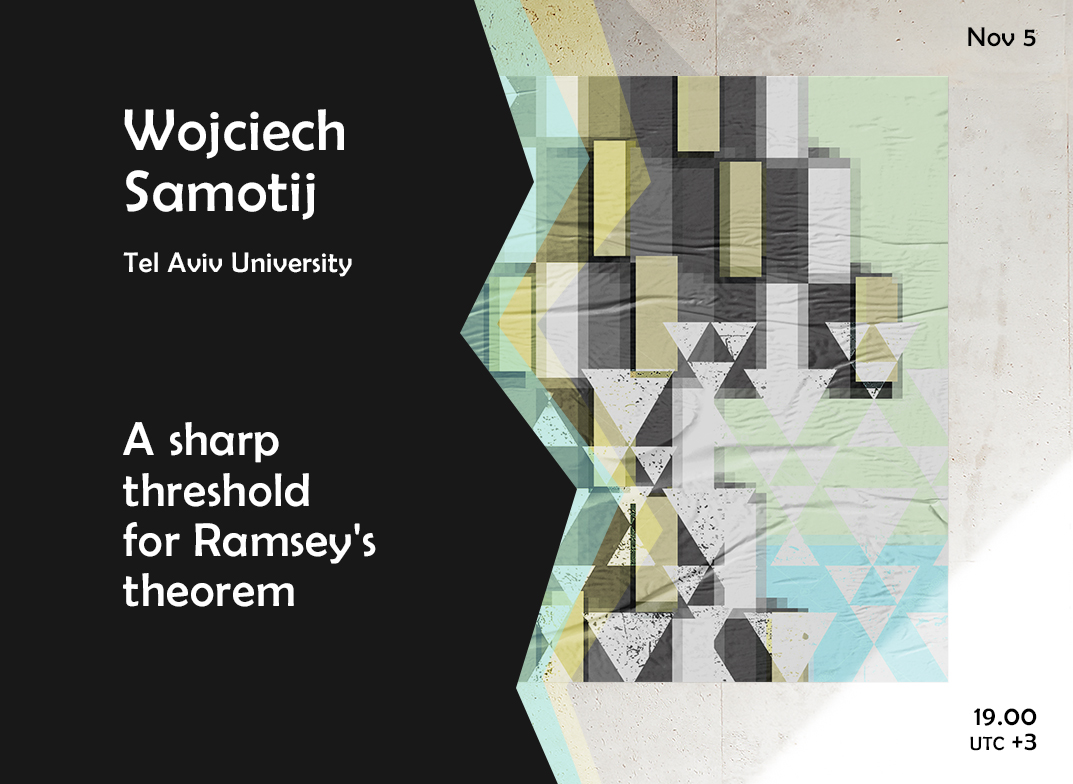# Wojciech Samotij “A sharp threshold for Ramsey’s theorem” | Big SeminarNovember 5, 2020
19.00 MSK (UTC +3)

# Wojciech Samotij "A sharp threshold for Ramsey's theorem"

Wojciech Samotij from Tel Aviv University will give the talk "A sharp threshold for Ramsey's theorem" on the labs' Big Seminar.

The talk will be held in zoom
Meeting ID: 279-059-822
Password: first 6 decimal places of $\pi$ after the decimal point

You can also write to Alexander Polyanskii (alexander.polyanskii@yandex.ru) or to Maksim Zhukovskii (zhukmax@gmail.com) if you want to be added to mailing list.

Abstract:

Given graphs $G$ and $H$ and an integer $r \ge 2$, we write $G \to (H)_r$ if every $r$-colouring of the edges of $G$ contains contains a monochromatic copy of $H$. It follows from Ramsey's theorem that, when $n$ is sufficiently large, $G \to (H)_r$ is a nontrivial, monotone property of subgraphs $G$ of $K_n$. The celebrated work of Rödl and Ruciński from the 1990s located the threshold for this property in the binomial random graph $G_{n,p}$ for all $H$ and $r$. We prove that this threshold is sharp when $H$ is a clique or a cycle, for every number of colours $r$; this extends earlier results of Friedgut, Rödl, Ruciński, and Tetali and of Schacht and Schulenburg.

This is joint work with Ehud Friedgut, Eden Kuperwasser, and Mathias Schacht.

Slides

## Watch the video:

Everyone is invited to attend. The language of the lecture is English. The event is aimed at master and graduate students, as well as researchers in the field of combinatorics.